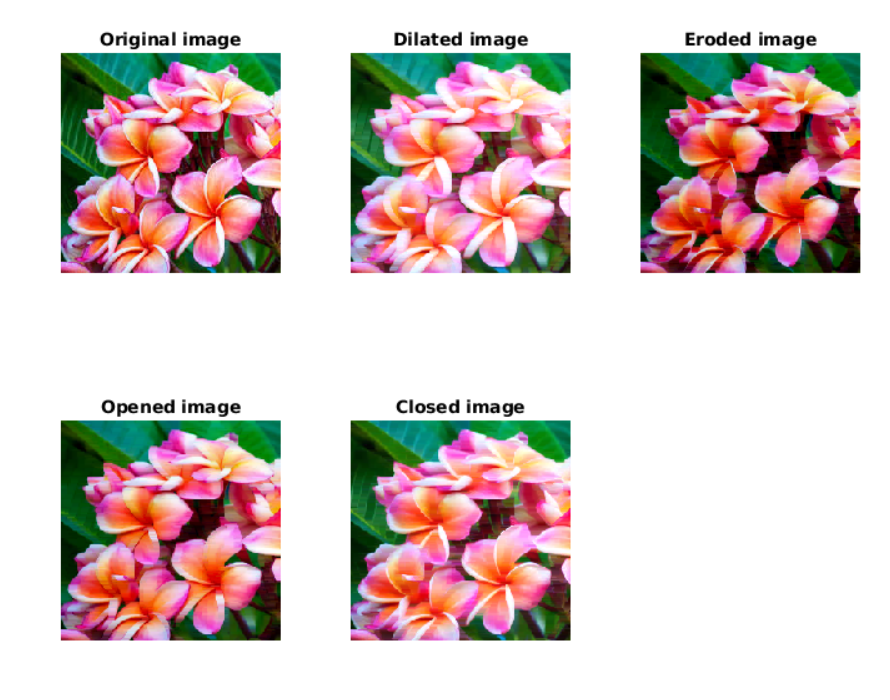Related Articles
Morphological operations in MATLAB
• Last Updated : 17 Aug, 2020

Morphological Operations is a broad set of image processing operations that process digital images based on their shapes. In a morphological operation, each image pixel is corresponding to the value of other pixel in its neighborhood. By choosing the shape and size of the neighborhood pixel, you can construct a morphological operation that is sensitive to specific shapes in the input image. Morphological operations apply a structuring element called strel in Matlab, to an input image, creating an output image of the same size.

Types of Morphological operations:

• Dilation: Dilation adds pixels on the object boundaries.
• Erosion: Erosion removes pixels on object boundaries.
• Open: The opening operation erodes an image and then dilates the eroded image, using the same structuring element for both operations.
• Close: The closing operation dilates an image and then erodes the dilated image, using the same structuring element for both operations.

The number of pixels added or removed from the object in an image depends on the shape and size of the structuring element used to process the image. In the morphological dilation and erosion operations, the state of any given pixel in the output image is determined by applying a rule to the corresponding pixel and its neighbors in the input image. The rule used to process the pixels defines the morphological operation as a dilation or an erosion.

Below is the Matlab code for Morphological operations:

 `# Importing the image ` `I = imread(``"flowers.jpg"``);  ` `subplot(2, 3, 1),   ` `imshow(I);  ` `title(``"Original image"``);  ` ` `  `% Dilated Image  ` `se = strel(``"line"``, 7, 7);  ` `dilate = imdilate(I, se);  ` `subplot(2, 3, 2),   ` `imshow(dilate);  ` `title(``"Dilated image"``);  ` ` `  `% Eroded image  ` `erode = imerode(I, se);  ` `subplot(2, 3, 3),   ` `imshow(erode);  ` `title(``"Eroded image"``);  ` ` `  `% Opened image  ` `open = imopen(I, se);  ` `subplot(2, 3, 4),   ` `imshow(open);  ` `title(``"Opened image"``);  ` ` `  `% Closed image  ` `close = imclose(I, se);  ` `subplot(2, 3, 5),   ` `imshow(close);  ` `title(``"Closed image"``);  `Attention reader! Don’t stop learning now. Get hold of all the important CS Theory concepts for SDE interviews with the CS Theory Course at a student-friendly price and become industry ready.

My Personal Notes arrow_drop_up
Recommended Articles
Page :